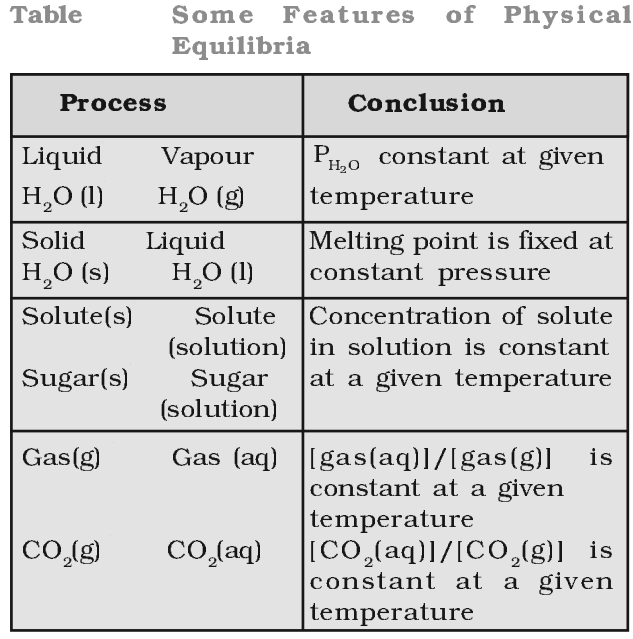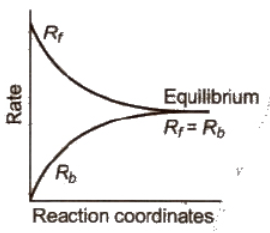When rate of formation of a product in a process is in competition with rate of formation of reactants, the state is then named as “Equilibrium state”.

Equilibrium may be classified as:

## Physical Equilibrium

Equilibrium set up in physical processes like evaporation of water, melting of solids, dissolution of solutes, etc., is called physical equilibrium, e.g., Ice ⇔ Water

At equilibrium,

Rate of melting of ice = Rate of freezing of water## Chemical Equilibrium

If a reversible reaction is carried out in a closed vessel, a stage is attained where the speed of the forward reaction equals the speed of the backward reaction. It corresponds to chemical equilibrium.

At equilibrium,

Rate of forward reaction = Rate of backward reaction## Characteristics of Chemical Equilibrium

• At equilibrium, the concentration of each of the reactants and the products becomes constant
• At equilibrium, the rate of forward reaction becomes equal to the rate of backward reaction and hence the equilibrium is dynamic in nature.
• A chemical equilibrium can be established only if none of the products is allowed to escape out or separate out as a solid.
• Chemical equilibrium can be attained from either direction, i.e., from the direction of the reactants as well as from the direction of the products.

Click on a star to rate it!

Average rating 0 / 5. Vote count: 0

No votes so far! Be the first to rate this post.

As you found this post useful...